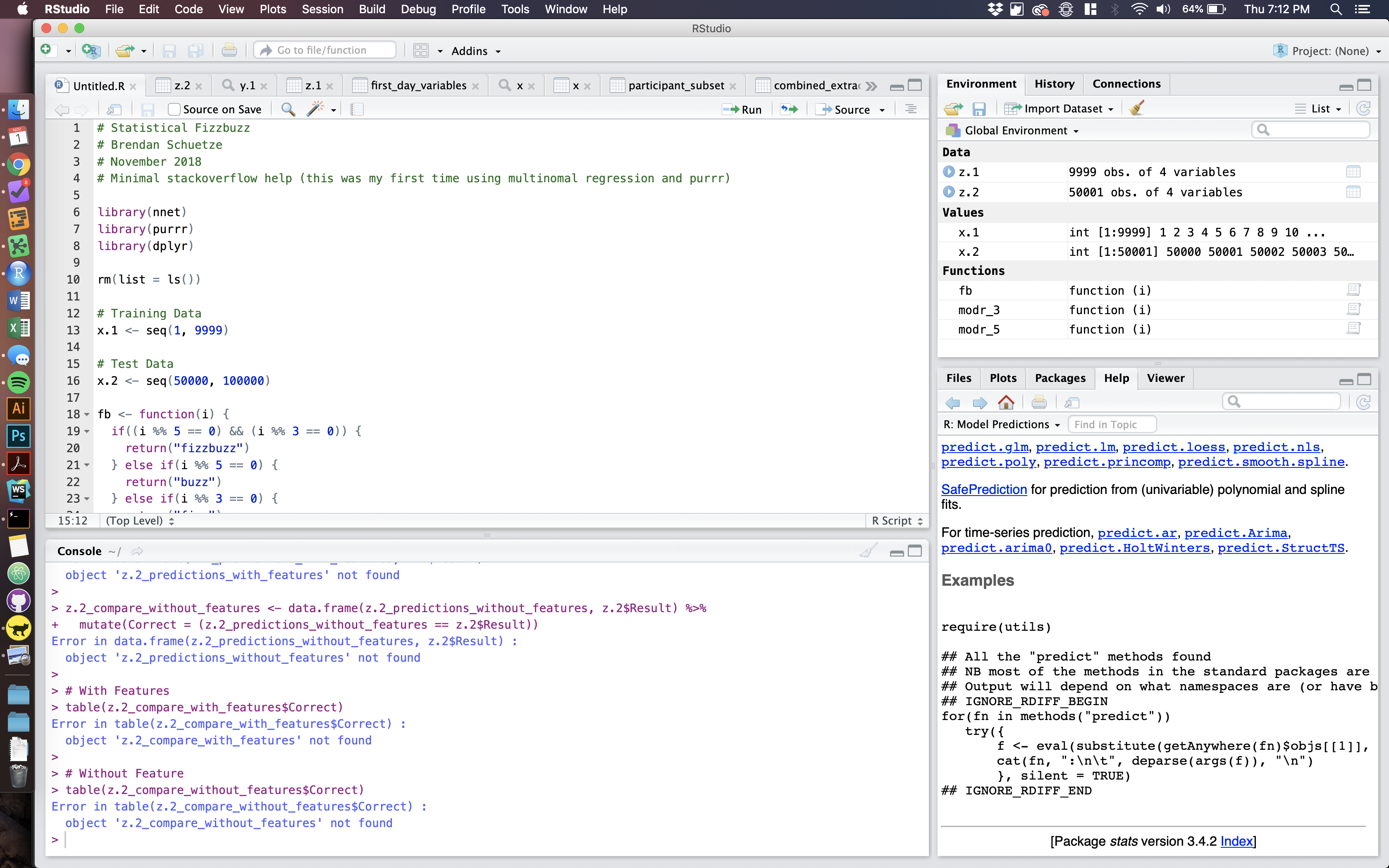# Statistical Fizzbuzz:

## My Take on the Classic CS Interview Question

Today, I was having fun in R with the classic computer science interview problem, "FizzBuzz." FizzBuzz (alternatively "Fizz Buzz") is a simple task – often used to teach kids about division. Given a list of integers, run through each one and determine if they are divisible by three and/or five. If the number is divisible by three, return "Fizz." If it is divisible by five, return "Buzz." If divisible by both three and five, return "FizzBuzz."### Background

This interview question is easy, if you know the modulo operator in your language of choice. Modulo division returns the remainder that would result from long division. In most languages modulo division is represented by the % operator. In R, you can perform modulo division using %%, as in 5 %% 3 will return 2

Although FizzBuzz is a fun way to learn the basics of a new programming language, I would consider myself an intermediate to advanced R programmer. I can usually figure out any data cleaning problem given to me in R, though my solution may not always be the prettiest. As such, I was thinking that it would be fun to teach a statistical model how to complete FizzBuzz.

### The Statistics

In order to perform FizzBuzz you need to use some form of classification algorithm that can handle more than two results. Most of my classification experience has dealt with binary outcomes, so I thought this would be a fun exercise to become acquainted with a new technique. After a short period of googling, I decided upon the "nnet" implementation of multinomial logistic regression.

Unfortunately (albeit forgivably) I found that the default nnet algorithm was not able to adequately learn the relationship between the inputs and the results when given only the input as the predictor. As a result, I had to do a little feature engineering. In this case, feature engineering meant calculating the modular division of each input by three and five and using the results as predictors.

If you run the code below, you can see that the new model with the two additional predictors can become 100 percent accurate if given enough training trials (approximately 2500 training trials are necessary depending on the range of numbers you are trying to learn). The naive algorithm never seems to get above 50 percent accuracy, which is better than chance, but not nearly 100 percent.

Overall this was a fun exercise. R's package library made this exceptionally easy to implement and test. Although I didn't see any examples of FizzBuss using a multinomial regression in R, FizzBuzz has been accomplished in many different ways. So, if you are interested in other takes on FizzBuzz and machine learning, you are in luck.  

### The Code

Someday, I will learn how to host Jupyter notebooks using GitHub, but alas today was not that day.